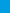# GED Mathematical Reasoning Prep 2019 A Comprehensive Review and Ultimate Guide to the GED Math TestGED Mathematical Reasoning Prep 2019 provides students with the confidence and math skills they need to succeed on the GED Math, building a solid foundation of basic Math topics with abundant exercises for each topic. It is designed to address the needs of GED test takers who must have a working knowledge of basic Math.\$12.99\$5.99
-54% only \$5.99

The Only Book You’ll Ever Need to ACE the GED Math Exam!

GED Mathematical Reasoning Prep 2019 provides students with the confidence and math skills they need to succeed on the GED Math, building a solid foundation of basic Math topics with abundant exercises for each topic. It is designed to address the needs of GED test takers who must have a working knowledge of basic Math.

This comprehensive book with over 2,500 sample questions and 2 complete GED tests is all you need to fully prepare for the GED Math. It will help you learn everything you need to ace the math section of the GED.

There are more than 2,500 Math problems with answers in this book.

Effortless Math unique study program provides you with an in-depth focus on the math portion of the exam, helping you master the math skills that students find the most troublesome.

This book contains most common sample questions that are most likely to appear in the mathematics section of the GED.

Inside the pages of this comprehensive GED Math book, students can learn basic math operations in a structured manner with a complete study program to help them understand essential math skills. It also has many exciting features, including:

• Dynamic design and easy-to-follow activities
• A fun, interactive and concrete learning process
• Targeted, skill-building practices
• Fun exercises that build confidence
• Math topics are grouped by category, so you can focus on the topics you struggle on
• All solutions for the exercises are included, so you will always find the answers
• 2 Complete GED Math Practice Tests that reflect the format and question types on GED

GED Mathematical Reasoning Prep 2019 is an incredibly useful tool for those who want to review all topics being covered on the GED test. It efficiently and effectively reinforces learning outcomes through engaging questions and repeated practice, helping you to quickly master basic Math skills.

Get a copy today and see how fast you will prepare for the test with the GED Math Workbook!